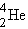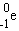Reactions and Changes

# Nuclear Chemistry: An Introduction

by Anthony Carpi, Ph.D.

Traditional chemical reactions occur as a result of the interaction between valence electrons around an atom's nucleus (see our Chemical Reactions module for more information). In 1896, Henri Becquerel expanded the field of chemistry to include nuclear changes when he discovered that uranium emitted radiation. Soon after Becquerel's discovery, Marie Sklodowska Curie began studying radioactivity and completed much of the pioneering work on nuclear changes. Curie found that radiation was proportional to the amount of radioactive element present, and she proposed that radiation was a property of atoms (as opposed to a chemical property of a compound). Marie Curie was the first woman to win a Nobel Prize and the first person to win two (the first, shared with her husband Pierre Curie and Henri Becquerel for discovering radioactivity; the second for discovering the radioactive elements radium and polonium).

In 1902, Frederick Soddy proposed the theory that "radioactivity is the result of a natural change of an isotope of one element into an isotope of a different element." Nuclear reactions involve changes in particles in an atom's nucleus and thus cause a change in the atom itself. All elements heavier than bismuth (Bi) (and some lighter) exhibit natural radioactivity and thus can "decay" into lighter elements. Unlike normal chemical reactions that form molecules, nuclear reactions result in the transmutation of one element into a different isotope or a different element altogether (remember that the number of protons in an atom defines the element, so a change in protons results in a change in the atom). There are three common types of radiation and nuclear changes:

Alpha Radiation (α) is the emission of an alpha particle from an atom's nucleus. An α particle contains two protons and two neutrons (and is similar to a He nucleus:). When an atom emits an a particle, the atom's atomic mass will decrease by four units (because two protons and two neutrons are lost) and the atomic number (z) will decrease by two units. The element is said to "transmutate" into another element that is two z units smaller. An example of an α transmutation takes place when uranium decays into the element thorium (Th) by emitting an alpha particle, as depicted in the following equation:

(Note: in nuclear chemistry, element symbols are traditionally preceded by their atomic weight [upper left] and atomic number [lower left].)

Beta Radiation (β) is the transmutation of a neutron into a proton and an electron (followed by the emission of the electron from the atom's nucleus:). When an atom emits a β particle, the atom's mass will not change (since there is no change in the total number of nuclear particles); however, the atomic number will increase by one (because the neutron transmutated into an additional proton). An example of this is the decay of the isotope of carbon called carbon-14 into the element nitrogen:

Gamma Radiation (γ) involves the emission of electromagnetic energy (similar to light energy) from an atom's nucleus. No particles are emitted during gamma radiation, and thus gamma radiation does not itself cause the transmutation of atoms; however, γ radiation is often emitted during, and simultaneous to, α or β radioactive decay. X-rays, emitted during the beta decay of cobalt-60, are a common example of gamma radiation.

Comprehension Checkpoint

Radiation can result in an atom having a different atomic number.

## Half-life

Radioactive decay proceeds according to a principle called the half-life. The half-life (T½) is the amount of time necessary for one-half of the radioactive material to decay. For example, the radioactive element bismuth (210Bi) can undergo alpha decay to form the element thallium (206Tl) with a reaction half-life equal to five days. If we begin an experiment starting with 100 g of bismuth in a sealed lead container, after five days we will have 50 g of bismuth and 50 g of thallium in the jar. After another five days (ten from the starting point), one-half of the remaining bismuth will decay and we will be left with 25 g of bismuth and 75 g of thallium in the jar. As illustrated, the reaction proceeds in halves, with half of whatever is left of the radioactive element decaying every half-life period.

The fraction of parent material that remains after radioactive decay can be calculated using the equation:

 Fraction remaining = 1  2n (where n = # half-lives elapsed)

The amount of a radioactive material that remains after a given number of half-lives is therefore:

 Amount remaining = Original amount * Fraction remaining

The decay reaction and T½ of a substance are specific to the isotope of the element undergoing radioactive decay. For example, Bi210 can undergo a decay to Tl206 with a T½of five days. Bi215, by comparison, undergoes bdecay to Po215 with a T½ of 7.6 minutes, and Bi208 undergoes yet another mode of radioactive decay (called electron capture) with a T½ of 368,000 years!

Comprehension Checkpoint

All radioactive material decays at the same rate.

## Stimulated nuclear reactions

While many elements undergo radioactive decay naturally, nuclear reactions can also be stimulated artificially. Although these reactions also occur naturally, we are most familiar with them as stimulated reactions. There are two such types of nuclear reactions:

1) Nuclear fission: reactions in which an atom's nucleus splits into smaller parts, releasing a large amount of energy in the process. Most commonly this is done by "firing" a neutron at the nucleus of an atom. The energy of the neutron "bullet" causes the target element to split into two (or more) elements that are lighter than the parent atom.

During the fission of U235, three neutrons are released in addition to the two daughter products. If these released neutrons collide with nearby U235 nuclei, they can stimulate the fission of these atoms and start a self-sustaining nuclear chain reaction. This chain reaction is the basis of nuclear power. As uranium atoms continue to split, a significant amount of energy is released from the reaction. The heat released during this reaction is harvested and used to generate electrical energy.

Nuclear fusion: reactions in which two or more elements "fuse" together to form one larger element, releasing energy in the process. A good example is the fusion of two "heavy" isotopes of hydrogen (deuterium: H2 and tritium: H3) into the element helium.